CIE Set (2018-2020)

 1 (CIE $0606 / 2018 / \mathrm{w} / 21 / \mathrm{q} 1 \mathrm{l})$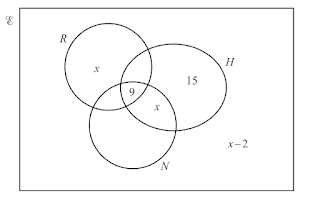There are 70 girls in a year group at a school. The Vemn diagram gives some information about the numbers of these girls who play rounders $(R)$, hockey $(H)$ and netball $(N)$ $$\mathrm{n}(R)=28 \quad \mathrm{n}(H)=38 \quad \mathrm{n}(N)=35$$ Find the value of $x$ and hence the number of girls who play netball only.

 2 (CIE $0606 / 2018 / \mathrm{w} / 22 / \mathrm{q}2$ )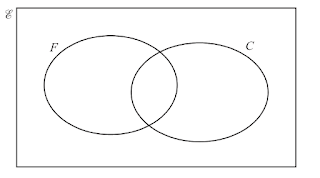There are 105 boys in a year group at a school. Some boys play football $(F)$ and some play cricket ( $C)$. $x$ boys play both football and cricket. The number of boys that play neither game is The same as The number of boys that play both. 40 boys play cricket. The number of boys that only play football is twice The number of boys that only play cricket. Complete the Venn diagram and find the value of $x$. 

 3 (CIE 0606/2018/w/23/q2) On each of the Venn diagrams below, shade the region indicated.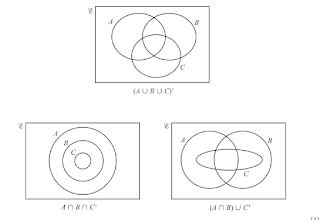4 (CIE 0606/2019/s/11/q1)
 (a) On the Venn diagrams below, shade the region indicated.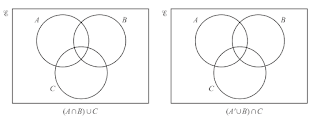(b) On the Venn diagram below, draw sets $P, Q$ and $R$ such that $$P \subset R, Q \subset R \text { and } P \cap Q=\emptyset$$ 

 5 (CIE 0606/2019/w/11/q1) Using set notation, describe the regions shaded on the Venn diagrams below.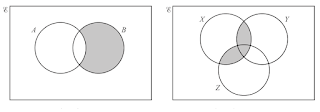6 (CIE $0606 / 2019 / \mathrm{m} / 12 / \mathrm{q} 1$ )
 (a) Given that \begin{aligned}&\mathcal{E}=\{x: 1 < x < 20\} \\& A=\{\text { multiples of } 3\} \\& B=\{\text { multiples of } 4\}\end{aligned} find (i) $\mathrm{n}(A)$,  (ii) $\mathrm{n}(A \cap B)$ $$
 (b) On the Venn diagram below, draw the sets $P, Q$ and $R$ such that $P \subset Q$ and $Q \cap R=\varnothing$.
 (c) Using set notation, describe the shaded areas shown in the Venn diagrams below.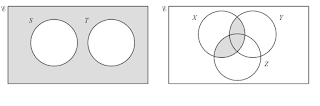7 (CIE 0606/2019/s/12/q1)
 (a) On the Venn diagrams below, shade the region indicated.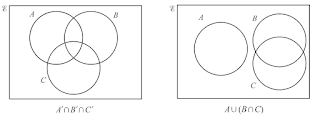$$
 (b) $$\left\{\begin{array}{l}\mathcal{E}=\left\{x: 0^{\circ} \leqslant x \leqslant 360^{\circ}\right\} \\P=\{x: \cos 2 x=0.5\} \\Q=\{x: \sin x=0.5\}\end{array}\right.$$ Find $P \cap Q$.$$

 8 (CIE 0606/2019/s/13/q1) Describe, using set notation, the relationship between the sets shown in each of the Venn diagrams below.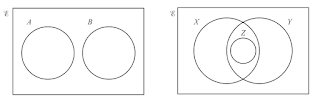$$

9 (CIE 0606/2019/w/13/q1)
In a group of 145 students, the numbers studying mathematics, physics and chemistry are given below. All students study at least one of the three subjects. $x$ students study all 3 subjects
24 students study both mathematics and chemistry
23 students study both physics and chemistry
28 students study both mathematies and physics
50 students study chemistry
75 students study physics
80 students study mathematics
 (i) Using the Venn diagram, find the value of $x$.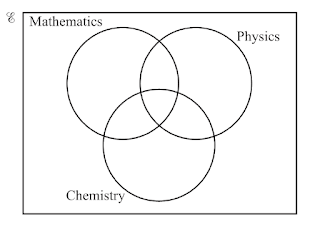(ii) Find the number of students who study mathematics only. $$

 10 (CIE 0606/2019/w/22/q1) On each of the Venn diagrams below, shade the region indicated.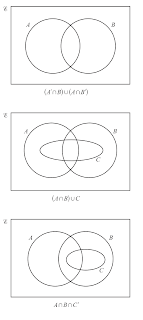1. $x=8$
2. $x=15$
3. 
4. 
5. $A'\cap B,(X\cap Y)\cup (X\cap Z)$
6. (a)(i) 6 (ii) 1 (b) (c) $S'\cap T', (X\cap Y)\cup (X\cap Z)$
7. (a) (b)$P\cap Q=\{30^{\circ},150^{\circ}\}$
8. $A\cap B=\emptyset,Z\subset (X\cap Y)$
9. (i) $x=15$ (ii) 43
10.